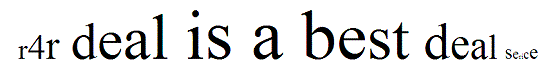# JavaScript Tutorial

Animation In JavaScript
 Previous Home Next

javascript provide Animation which is based on a phenomenon called persistence of vision The concept of animating is moving of an object in Javascript .

The object that is being animated is changed over a number of steps set at a certain interval over a certain period of time at each step the object may be changed in any number of ways

### Example :

```<Html>
<script>
var theText = "r4r deal is a best deal service";
function nextSize(i,incMethod,textLength)
{
if (incMethod == 1) return (85*Math.abs( Math.sin(i/(textLength/3.14))) );
if (incMethod == 2) return (275*Math.abs( Math.cos(i/(textLength/3.14))));
}

function sizeCycle(text,method,dis)
{
output = "";
for (i = 0; i < text.length; i++)
{
size = parseInt(nextSize(i +dis,method,text.length));
output += "<font style='font-size: "
+ size +"pt'>" +text.substring(i,i+
1)+
"</font>";
}
theDiv.innerHTML = output;
}

function doWave(n)
{
sizeCycle(theText,1,n);
if (n > theText.length) {n=0}
setTimeout ("doWave("
+ (n+1) + ")",
50);
}
</script>